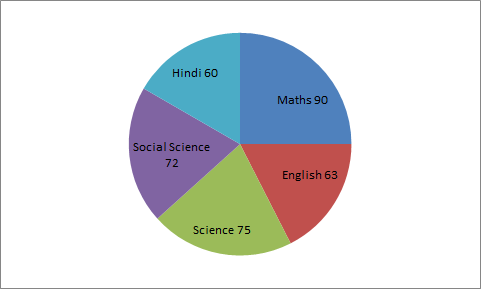# Data Interpretation - Pie Chart

>>>>>>>>Pie Chart

Instructions:

The following pie chart shows the marks obtained by a student in an examination, who scored 540 marks in allStudy the graph and answer the questions 31-33  given below

he subject in which the student scored 108 marks is

• A

Science• B

Hindi• C

English• D

Social Science• Option : D
• Explanation :

Central angle for 108 marks = (108/540)*360 = 72o

So, the student scored 108 marks in Social Science.

Instructions:

The following pie chart shows the marks obtained by a student in an examination, who scored 540 marks in allStudy the graph and answer the questions 31-33  given below

• A

Social Science• B

Hindi• C

English• D

Science• Option : B
• Explanation : Central angle for

16 2/3% = [50/(3*100)]*360 = 60o

So, he scored 16 2/3% marks in Hindi

Instructions:

The following pie chart shows the marks obtained by a student in an examination, who scored 540 marks in allStudy the graph and answer the questions 31-33  given below

• A

90• B

72• C

85• D

61• Option : A
• Explanation : Marks scored in Hindi and Mathematics

= [((60/360)*540) + ((90/360)*540)] = 225

• A• B• A• BRelated Quiz.
Pie Chart